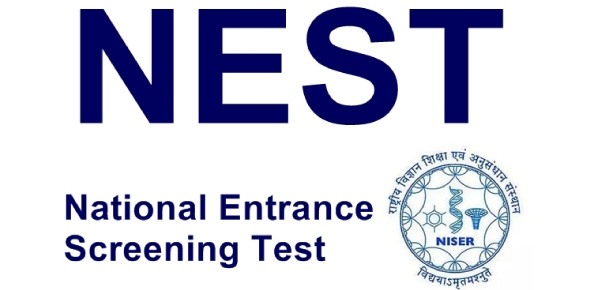# NEST Exam: MCQ Quiz!

Approved & Edited by ProProfs Editorial Team
The editorial team at ProProfs Quizzes consists of a select group of subject experts, trivia writers, and quiz masters who have authored over 10,000 quizzes taken by more than 100 million users. This team includes our in-house seasoned quiz moderators and subject matter experts. Our editorial experts, spread across the world, are rigorously trained using our comprehensive guidelines to ensure that you receive the highest quality quizzes.
| By Cripstwick
C
Cripstwick
Community Contributor
Quizzes Created: 693 | Total Attempts: 698,597
Questions: 10 | Attempts: 59Settings• 1.

### For admission to a five-year integrated MSc program in Basic Science, NEST is...

• A.

Optional

• B.

Compulsory

• C.

Depends on the school

• D.

None of the above

B. Compulsory
Explanation
NEST is compulsory for admission to a five-year integrated MSc program in Basic Science. This means that all applicants must take the NEST exam in order to be considered for admission into the program. It is not optional and does not depend on the school.

Rate this question:

• 2.

### When will NEST online application open?

• A.

2020

• B.

2019

• C.

2018

• D.

None of the above

C. 2018
Explanation
The correct answer is 2018. This means that the NEST online application opened in 2018.

Rate this question:

• 3.

### The examination always starts by...

• A.

11:45 AM

• B.

10:00 AM

• C.

10:30 AM

• D.

11:00 AM

B. 10:00 AM
Explanation
The correct answer is 10:00 AM because it is the only option that is a reasonable starting time for an examination. 11:45 AM is too late in the morning and would not leave enough time for students to complete the exam. 10:30 AM and 11:00 AM are also later starting times and would not align with typical examination schedules. Therefore, 10:00 AM is the most logical and appropriate choice for the start time of the examination.

Rate this question:

• 4.

### Which is one of the NEST rules?

• A.

Candidates should be at the examination centre half an half before the examination

• B.

Candidates should not eat

• C.

Candidates should ten minutes late after the examination

• D.

Candidates should not come

A. Candidates should be at the examination centre half an half before the examination
• 5.

### The question paper consists of how many sections?

• A.

Five sections

• B.

Two sections

• C.

Nine sections

• D.

Three sections

A. Five sections
Explanation
The question is asking about the number of sections in the question paper. The correct answer is "Five sections." This means that the question paper is divided into five different sections.

Rate this question:

• 6.

### The second section consists of how many subjects?

• A.

Four subjects

• B.

Two subjects

• C.

Three subjects

• D.

Six subjects

A. Four subjects
Explanation
The second section consists of four subjects.

Rate this question:

• 7.

### How many marks does Section A carry?

• A.

35 marks

• B.

10 marks

• C.

30 marks

• D.

None of the above

C. 30 marks
Explanation
Section A carries 30 marks.

Rate this question:

• 8.

• A.

September

• B.

November

• C.

April

• D.

July

C. April
Explanation

Rate this question:

• 9.

### Since the examination started, the number of candidates has...

• A.

Increased

• B.

Decreased

• C.

Remained constant

• D.

I don't know

A. Increased
Explanation
The correct answer is "Increased" because the statement mentions that "since the examination started," indicating a specific point in time. Therefore, it can be inferred that the number of candidates has grown or become larger over that period.

Rate this question:

• 10.

### Which is a subject offered?

• A.

Mathematics

• B.

History

• C.

Agricultural science

• D.

Computer studies

A. Mathematics
Explanation
Mathematics is a subject that deals with the study of numbers, quantity, and space. It involves the understanding and application of concepts such as algebra, geometry, calculus, and statistics. It is widely taught in schools and universities as it is considered fundamental to many other fields of study, including science, engineering, and finance. Therefore, the subject of Mathematics is indeed offered as a course of study.

Rate this question:

Related TopicsBack to top